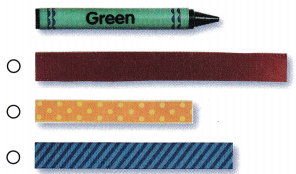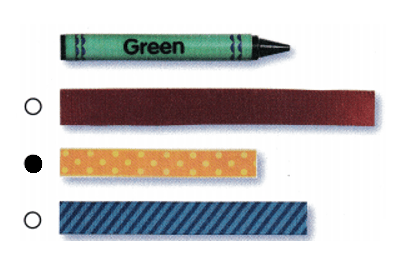Refer to our Texas Go Math Grade 1 Answer Key Pdf to score good marks in the exams. Test yourself by practicing the problems from Texas Go Math Grade 1 Module 17 Assessment Answer Key.

Vocabulary

Question 1.
Circle the piece of string that is the same length as the spoon. Explain how you got your answer.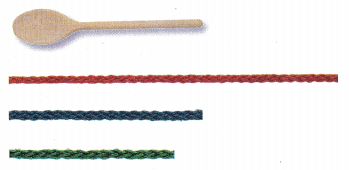Length of blue string is as same as the length of the spoon.Explanation:
Length of red string is more than the length of the spoon.
Length of blue string is equal to the length of the spoon.
Length of green string is less than the length of the spoon.

Concepts and Skills
Question 2.
Circle the ribbon that is about 2long. (TEKS 1.7.B, 1.7.D)Length of green ribbon is about the length of the 2.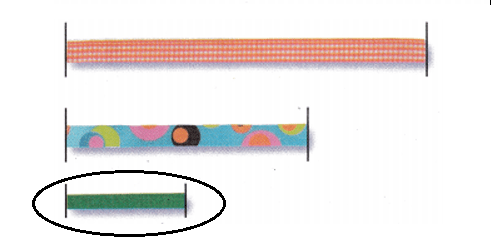Explanation:
Length of red ribbon is more than the length of the 2.
Length of blue ribbon is more than the length of the 2.
Length of green ribbon is about the length of the 2.

Question 3.
Draw a pencil that is about 5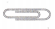long. (TEKS 1.7.B, 1.7.D)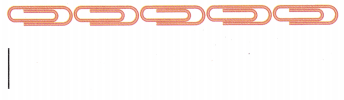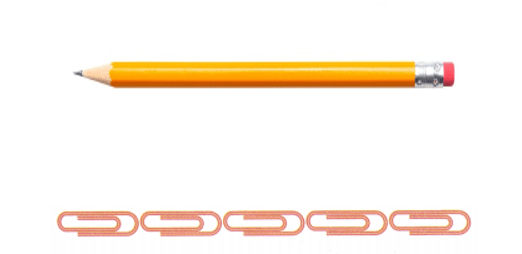Explanation:
Length of the pencil is drawn as same as the fivelong.

Question 4.
Which piece of yarn is longer (TEKS 1.7.A)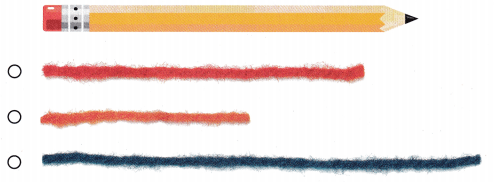Length of blue piece of yarn is longer than the length of the pencil.Explanation:
Length of red piece of yarn is same as the length of the pencil.
Length of orange piece of yarn is less than the length of the pencil.
Length of blue piece of yarn is more than the length of the pencil.

Question 5.
Useand real objects. Which object is about 3long? (TEKS 1.7.B, 1.7.D)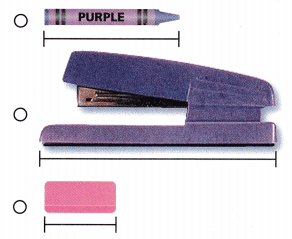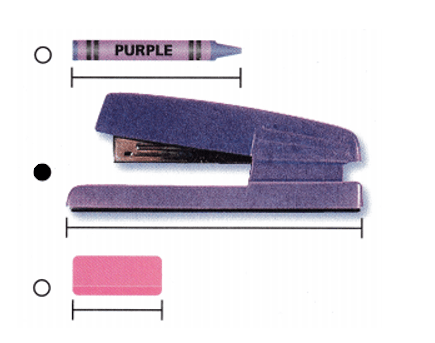Explanation:
Length of crayon is about the length of the 2.
Length of stapler is about the length of the 3.
Length of eraser  is about the length of the 1.

Question 6.
Which unit would you use the most of when measuring the same object? (TEKS 1.7.C )
(A)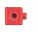(B)(C)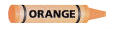String is generally used most for measuring the same object. String is good tool to measure the objects.
(B).

Explanation:
Block as a measuring tool is used when specifically referred to measure the objects.
String is good tool to measure the objects. It is generally used most for measuring the same object.
Crayon is not a measuring tool to measure the objects.

Question 7.
Which ribbon is shorter than the crayon? (TEKS 1.7.A)